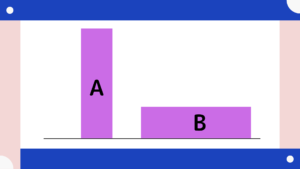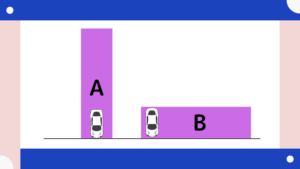# Depth of inheritance tax and land valuation, and land usability can reduce valuation.

A significant point in calculating inheritance taxes would be the valuation of assets.

For cash, 100 million yen is valued at 100 million yen.
However, how much would a 100 million yen piece of land actually be valued?

Naturally, there is a method of estimating roadside land prices.
However, this method makes it difficult to express the actual land condition only.

To reflect individual circumstances, the valuation will vary depending on the shape of the land.
In this article, we will review how the assessed value of land decreases with the depth of the land to consider land valuation for inheritance tax purposes.

## Usability of land

Suppose there is a piece of land in this shape.
Both are priced at 100 million yen.

Let’s assume that both have the same area.When comparing the two lots, the one that faces the road longer is more usable.
This is well understood by those who have constructed custom-built houses or buildings.

The ease of use of a lot with a broader frontage on the street is easier to understand when you consider parking space.
When you park one car, you can park it any way you want if it is wide enough to face the street, but if it is deep enough, it is less usable to park a car.This difference is represented in the rating.

## Depth Correction

### The deeper the depth, the lower the valuation of the land.

There is a depth correction ratio.
This concept considers the calculated and theoretical depth of the land, and if the depth is inevitably long, the valuation is lowered.

Depth” or “depth distance” is a significant indicator in inheritance tax and land valuation. This depth refers to the distance from where the land meets the road to the farthest point. However, physical depth is used in inheritance tax land valuation, and a unique measurement called “calculated depth distance” is used.

To give a specific example of the calculation, if the standard depth of land is 20 meters, the area of land to be assessed is 180 square meters, and the frontage is 5 meters, the result is 180 square meters divided by 5 meters, which is 36 meters. Comparing these two figures, the calculated depth distance is 20 meters because 20 meters is less than 36 meters.

You feel the need to conduct a field survey to evaluate the land.

### Depth to Frontage Length Ratio

Depth is not only longer but also considered deeper compared to frontage.

Frontage ÷ Depth

The idea is that the smaller the ratio, the lower the land valuation.
For example, let us assume the correction ratio is 6% lower.

If the inheritance tax assessment on a 100 million yen piece of land can be reduced by 6%,

100 million yen x 6% = 6,000,000 yen

The 50-100 million yen tax rate is 30%, so =1,800,000 yen would reduce the tax rate.
The amount of inheritance tax paid can vary depending on whether or not the valuation is done properly.

This is a simplified calculation so it may be a little more complicated than that.
However, I hope this has given you an idea.

## Reflecting Individual Circumstances in Valuation

It is crucial to reflect individual circumstances in the valuation of land.
In addition to the depth correction introduced in this article, many other valuation items should be reflected in the land.

The tax exemption points are

Thirty million yen + 6 million yen x number of persons

and you need to be careful not to get stuck with this amount.
It is where you should consider to reflect the detailed land valuation and saving inheritance tax as per the law.

Even if you file a tax return, an explanation is necessary. If you are in doubt, please get in touch with us.